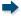Probability & Statistics - Maplesoft Books - Maple Books, Maple Resources and Math BooksHome : Maplesoft Books : Probability & StatisticsProbability & Statistics Search
 Maple: A Primer Bernard V Liengme 2019 Calculus | Computer Algebra | Differential Equations | Linear Algebra | Physics | Probability & Statistics | Recent Releases
 Advanced Problem Solving with Maple: A First Course William P. Fox William C. Bauldry 2019 Differential Equations | Mathematical Modeling | Optimization | Probability & Statistics | Programming | Recent Releases
 Stochastik für Dummies Christoph Maas 2013 Probability & Statistics
 Informal Introduction to Stochastic Processes with Maple Jan Vrbik, Paul Vrbik 2012 Computer Algebra | Probability & Statistics
 A Modern Theory of Random Variation: With Applications in Stochastic Calculus, Financial Mathematics, and Feynman Integration Patrick Muldowney 2012 Calculus | Probability & Statistics
 1 2 3 4Page 1 of 4
 Do you know of a book that should be included in our list? Tell us about it!
 Title/Author: Category: Any Category Abstract Algebra Biology Calculus Chemistry Complex Analysis Computer Algebra Computer Science Conference Proceedings Courseware Cryptography Differential Equations Differential Geometry Dynamical Systems Economics Engineering Games Linear Algebra Maple Manuals Mathematical Modeling Number Theory Numerical Analysis Operations Research Optimization Physics Pre-Calculus Probability & Statistics Programming Recent Releases Vector Calculus Language: Any Language English French German Spanish Japanese Dutch Russian Swedish Polish Persian ChineseAbstract Algebra Biology Calculus Chemistry Complex Analysis Computer Algebra Computer Science Conference Proceedings Courseware Cryptography Differential Equations Differential Geometry Dynamical Systems Economics Engineering Games Linear Algebra Maple Manuals Mathematical Modeling Number Theory Numerical Analysis Operations Research Optimization Physics Pre-Calculus Probability & Statistics Programming Recent Releases Vector Calculus